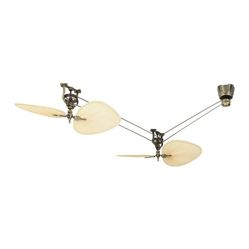# ceil ceilf ceill

ceil(+2.4) = +3.0 ceil(-2.4) = -2.0 ceil(-0.0) = -0.0 ceil(-Inf) = -inf

This function (for double argument) behaves as if (except for the freedom to not raiseFE_INEXACT) implemented by

Trigonometric and hyperbolic functions

Errors are reported as specified inmath_errhandling.

Nearest integer floating point operations

7.22 Type-generic math tgmath.h (p: 335-337)

The largest representable floating-point values are exact integers in all standard floating-point formats, so this function never overflows on its own; however the result may overflow any integer type (includingintmax_t), when stored in an integer variable.

7.12.9.1 The ceil functions (p: 231-232)

7.25 Type-generic math tgmath.h (p: 373-375)

7.12.9.1 The ceil functions (p: 251)

If the implementation supports IEEE floating-point arithmetic (IEC 60559),

computes largest integer not greater than the given value

F.10.6.1 The ceil functions (p: 526)

C89/C90 standard (ISO/IEC 9899:1990):

F.9.6.1 The ceil functions (p: 462-463)

FE_INEXACTmay be (but isnt required to be) raised when rounding a non-integer finite value.

If no errors occur, the smallest integer value not less thanarg, that is⌈arg⌉, is returned.

Floating point manipulation functions

Computes the smallest integer value not less than